Next: Limits within Up: Limits within [-1,1] Previous: A recursive definition

### Recursive calls to compute the limits

Suppose we have a stream of nested intervals represented as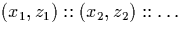, whose end points are represented as signed binary streams (section 3.5), and with the following property: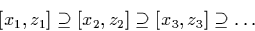We also have a function f which takes three signed binary stream x, y, and z as inputs, and outputs a signed binary stream representing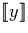by examining x and z, determining common digits from them if it can using the method described above, and then using y directly to determine the rest of the output.

We can now compute the signed binary stream represented by the stream of nested intervals y as the result of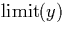, where the function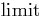function is defined as: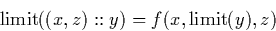Martin Escardo
5/11/2000